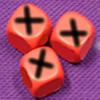Resource Title search

Text Search of NRICH resource titles

Or find resource begining with:X Is 5 Squares

Age 7 to 11 Challenge Level:

Can you arrange 5 different digits (from 0 - 9) in the cross in the way described?X Marks the Spot

Age 11 to 14 Challenge Level:

When the number x 1 x x x is multiplied by 417 this gives the answer 9 x x x 0 5 7. Find the missing digits, each of which is represented by an "x" .X-dice

Age 16 to 18 Challenge Level:

Explore these X-dice with numbers other than 1 to 6 on their faces. Which one is best?XOXOXO

Age 14 to 16 Short Challenge Level:

6 tiles are placed in a row. What is the probability that no two adjacent tiles have the same letter on them?Xtra

Age 14 to 18 Challenge Level:

Find the sides of an equilateral triangle ABC where a trapezium BCPQ is drawn with BP=CQ=2 , PQ=1 and AP+AQ=sqrt7 . Note: there are 2 possible interpretations.